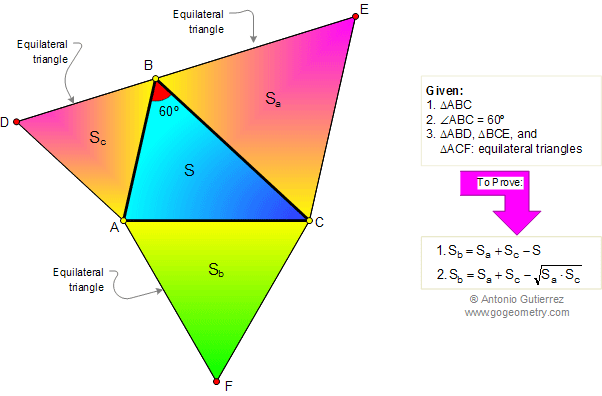Problem 211. 60 Degree Triangle, Equilateral triangles, Areas. Elearning.
 In the figure below, given a triangle ABC of area S, the measure of the angle ABC is 60 degree and ABD, BCE, and ACF are equilateral triangles of areas Sc, Sa, and Sb respectively. Prove that (1) Sb = Sa + Sc - S, and (2). View or post a solution.Home | Geometry | Search | Problems | Art | 211-220 | Area of a Triangle | Email | View or post a solution